# Why is the Standard Error Equal to Sigma Divided by the Square Root of n?Photo by Ken Treloar on Unsplash Every time I teach the Central Limit Theorem, I get questions from students on why we divide the population standard deviation, sigma, by the square root of the sample size to calculate the standard deviation of the sampling distribution which we call the standard error. Recall that the equation for the standard error iswhere σ is the population standard deviation and n is the sample size. One can find any number of precise “academic explanations” of why this is true, and I give my students links to those references. But I often get follow-up questions from students who look at those references and then ask for a simpler, clearer explanation that does not involve a lot of algebra. So, I am going to attempt one here and in the companion video.pixabay.com Let’s begin with our reliable dice as an example of a population. If we use the standard six-sided dice, and assuming the dice are fair, and the rolls are fair, then each face has an equal chance of coming up. Because there are six faces, 1,2,3,4,5,6, each face has 1/6 or 16.6666666…% chance of coming up. Let’s also assume that each roll of the dice is a sample of size n=1 since we just have one die. We know from basic probability that we need to look at the long-term when we are looking at empirical probabilities, so let us make 10,000 rolls of our single die. So, we then have 10,000 samples of sample size n = 1.
I am going to simulate doing 10,000 rolls of a single die using basic Excel. Here in Figure 1 is a screenshot of my worksheet with most of the rows hidden.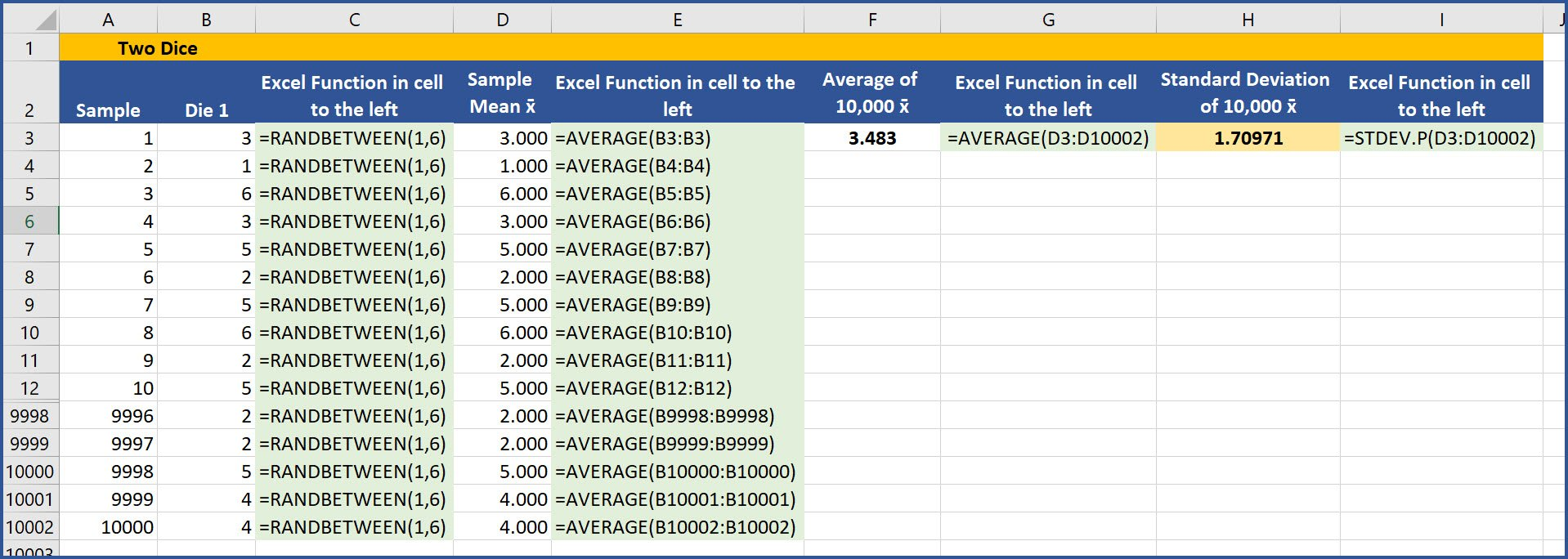Fig #1 In column A, I inserted a series from 1 to 10,000 in cells A3 to A10002. In column B, I used a standard Excel function, RANDBETWEEN, which returns a random integer between the limits shown (inclusive of 1 and 6) every time the worksheet is recalculated. I copied this formula down the range B3:B10002, as you can see in column C where I show the formulas in column B. When the worksheet is recalculated, each of those 10,000 cells calculates a new random value between 1 and 6, inclusive. Thus, we are simulating 10,000 rolls of a single die. Each cell in the range B3 to B10002 contains the face or value on that die. Each is a sample of size n = 1. Although calculating the mean, x-bar, of a sample of 1 is a bit trivial, I do that in column D using the Excel AVERAGE function. I copy that formula down the range D3:D10002, as you can see in column E where I show the formulas in column D. For the first sample, in cell D3 the average of the value on the single die, 3, is just 3.000. I am doing this to establish the format I will use for all the remaining sample sizes from 2 dice to 30 dice. In cell F3 using the Excel AVERAGE function over the cell range D3:D10002, I calculated the average of the sample means of the 10,000 dice rolls and that is 3.483, which is very close to the actual population mean of 3.500. [(1+2+3+4+5+6)/6] I also calculated the standard deviation of the means of those 10,000 samples in cell H3 using the Excel function STDEV.P over the cell range D3:D10002. That value is 1.70971, again very close the actual population standard deviation, σ, of 1.7078. [The standard deviation of 1,2,3,4,5,6 = 1.7078.] I could have simulated many more throws and would likely have gotten my “averages” closer to the theoretical values, but these 10,000 samples should be enough to give you the idea of how this works.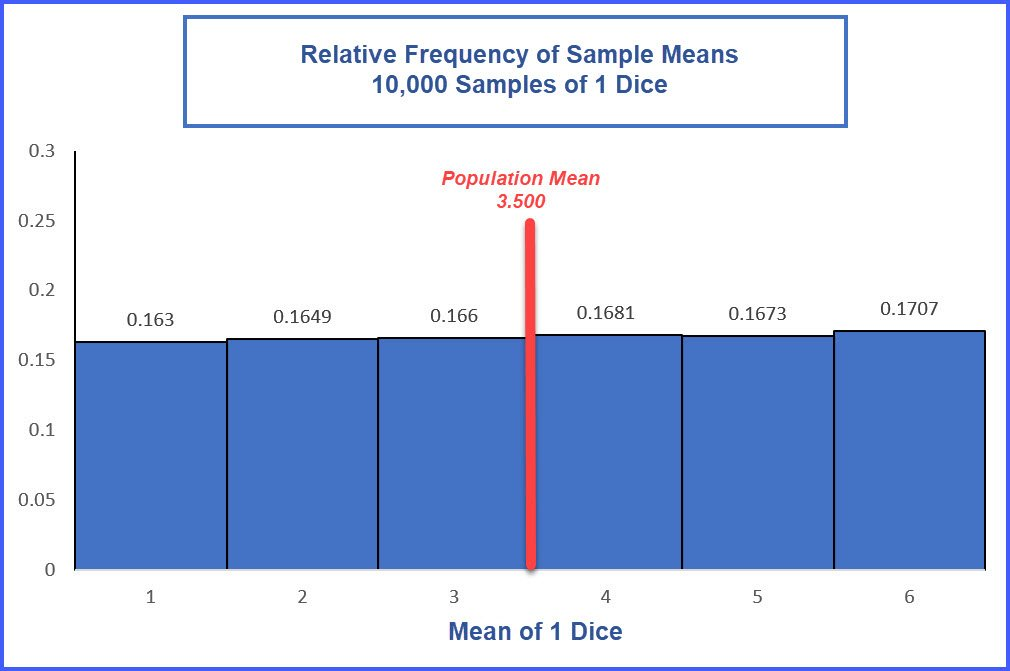Fig. #2

Figure 2 is the histogram showing the relative frequencies of the value of the 10,000 samples of size n = 1. They are not too different from the theoretical 16.666667%. I used just six bins because there are only six possible values for a throw of one die. I also show a line to represent the population mean of 3.5, though you cannot get a mean of 3.5 rolling just one die. The uniform spread of the values explains why the standard deviation sigma is relatively large compared to the mean.

Next, I will repeat the simulation using 2 dice, which is a sample of n = 2. Figure 3 is an image of the Excel worksheet constructed for a sample of 2 dice as was done for the first example of one die, a sample of n = 1. You can consider this to be the sampling distribution for two dice rolled 10,000 times. In column D, I have calculated the mean, x-bar, of the two dice, which is 2.500 (cell D3) for the first sample of n = 2. In column E, I show the formulas in the adjacent cells in column D. In Cell F3, I again calculated the average of the sample means, x-bar, for those 10,000 samples of n = 2 and that value is 3.496, again close to the theoretical 3.500 population mean. This is what we expect if we believe the Central Limit Theorem is true.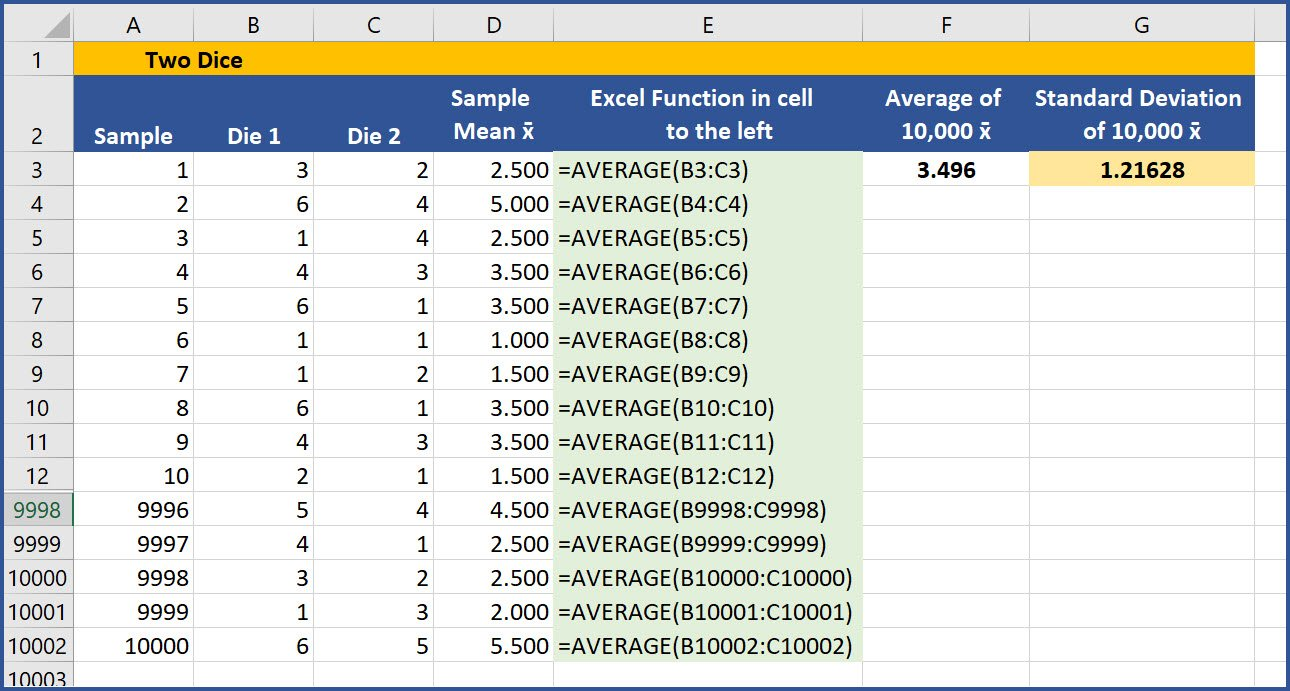Fig. #3 In Cell G3, I calculated the standard deviation of the sample averages, 1.21628. This is our standard error, the standard deviation of our sampling distribution for the mean of two dice. And you should notice it has decreased quite a bit from the standard deviation 1.7077 we had for a sample of size n = 1. Be sure to note the difference between the number of samples and the sample size, n. Here the number of samples is 10,000, but the sample size is just n = 2. This next graph (Figure 4)  is the relative frequency histogram of the average value of the two dice for the 10,000 samples. Note the distribution is beginning to resemble a “bell shape,” which is what should be expected if the Central Limit Theorem holds true. Also note, I increased the number of bins in the histogram to better display the distribution.Fig. #4 Now about 67% of the data falls in the middle 5 bins from 2.5 to 4.5. Because more of the values are closer to the population mean of 3.5, the standard deviation of the sampling distribution of sample means, the standard error, is 1.21628, which is much smaller than the population’s sigma of 1.7077 and also the standard deviation of our simulation using just 1 die of 1.70971. I repeated the Excel simulation for rolling 3 dice 10,000 times, and again for 4, 5, 6, … all the way to 30 dice being thrown 10,000 times, but I will not show all that information here in detail. I will use it for the very last part of this discussion.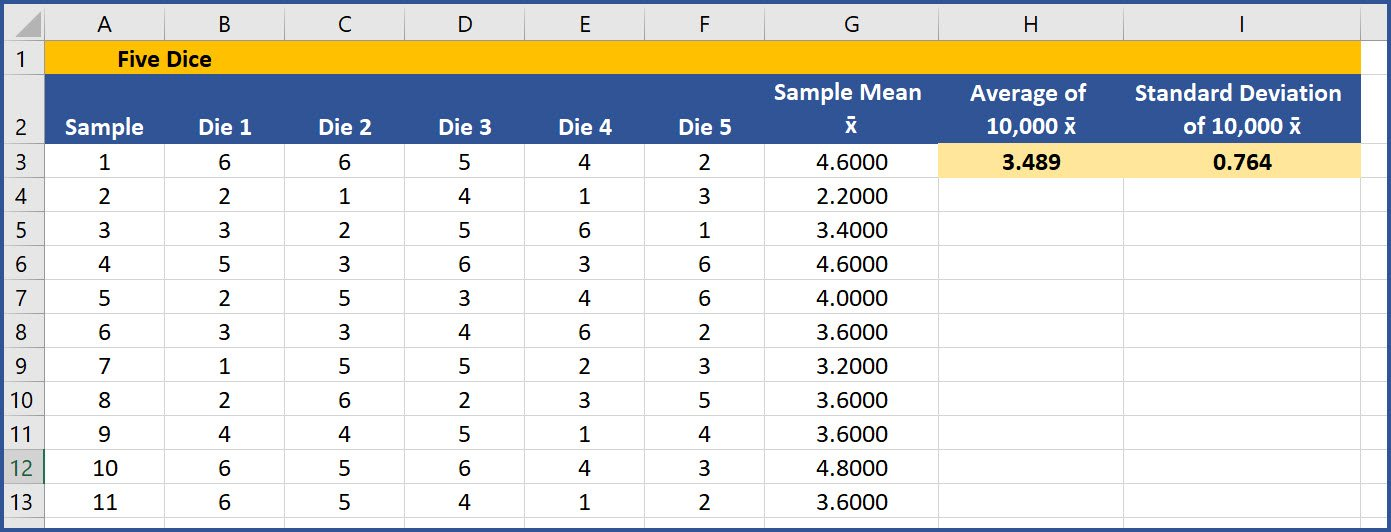Fig. #5 Figure 5 shows a portion of the Excel worksheet for 5 dice thrown 10,000 times. Remember these are 10,000 samples of size n = 5. In column G on this worksheet, I included a calculation of the average of the 5 dice using the Excel AVERAGE function again for each of the 10,000 rolls. The average of those 10,000 sample means is 3.489 in cell H5, again very close to the actual population mean of 3.500. And the standard error of these 10,000 samples of n = 5 is 0.764, calculated in cell I3. Recall that is the standard deviation of the sample means in column G, which is our sampling distribution of sample means.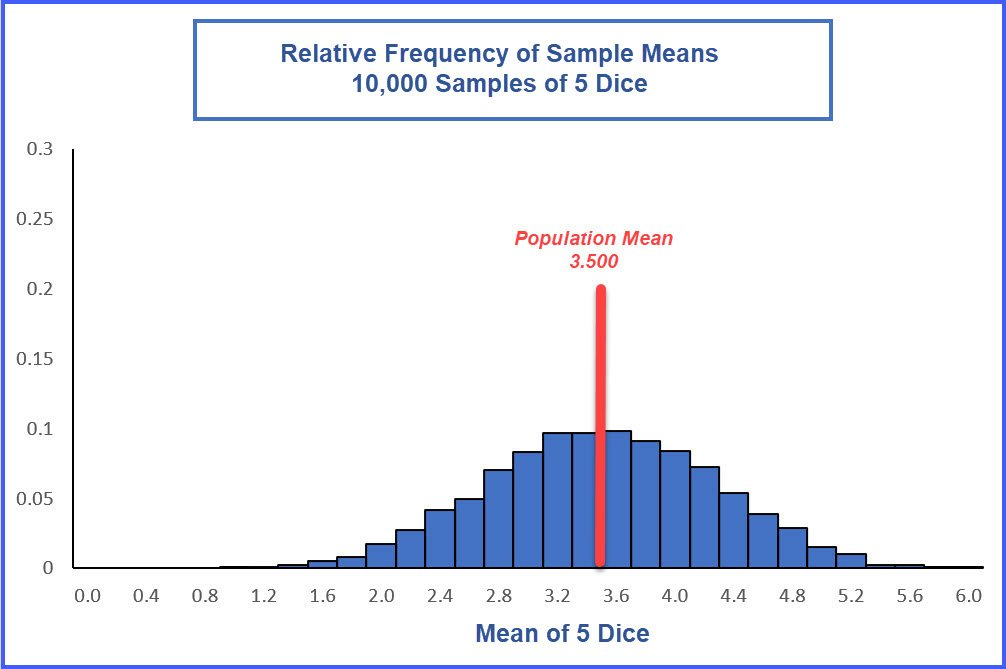Fig. #6 Figure 6 is the histogram for the relative frequencies of the sample mean for 5 dice thrown 10,000 times. It gets too crowded to show the individual bin frequencies on this chart, but you can see that the tails are slimmer, especially toward 0 since you cannot get less than 1 on a die and that means the mean for 5 dice must be at least 1, i.e. (1+1+1+1+1)/5 = 5/5 = 1. This parallels with the calculated standard error decreasing from that of the simulation with just two dice. As the sample size, n, increases, the standard error is decreasing. Repeating the simulation using 10 dice, 20 and 30 dice, we get these three relative frequency distributions for the 10,000 sample means for samples sizes n = 10 (Figure 7), n = 20 (Figure 8), and n = 30 (Figure 9):Fig. #7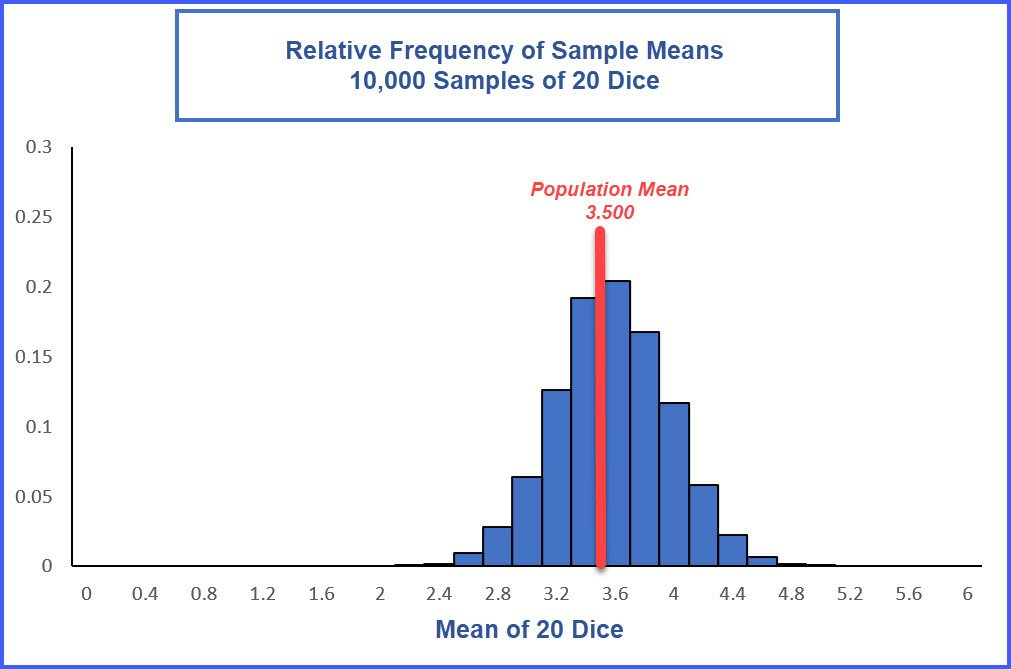Fig. #8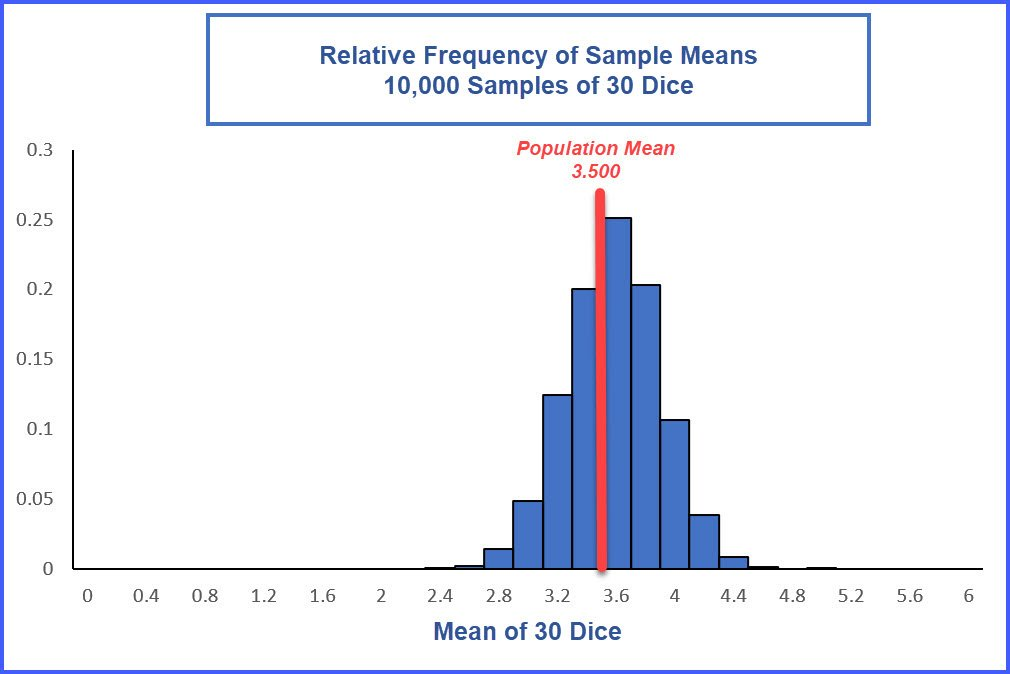Fig. #9 In the table in Figure 10, I have captured the relevant data from all the simulations I ran using this method in Excel. I also show a scatter plot of the data with the calculated standard error on the y-axis and the number of dice – the sample size n – on the x-axis. The orange dots are the individual data points for the 30 simulated standard errors.Fig. #10 Obviously, there is not a straight-line relationship here, if you recall plotting best-fit lines from geometry or algebra. Fortunately, Excel has a great tool that will let you select from lines that could fit your data. These are known as trend lines, or regression lines, and you will learn how to calculate them later in your intro stats course. For now, trust that Excel can find a “best fit” line for these data and calculate the equation that describes it.Fig. #11 Figure 11 shows a “Straight-line” or “Linear” best-fit trend line (small dots), which does not fit our data (large dots) all that well, as you would expect. The equation of this line Excel calculated is shown as y = – 0.0278*x + 0.9766. You can use that equation to predict values of y, the standard error, for different values on n. Of more interest now is the R2 value of 0.6345. R2 is the Coefficient of Determination, again a term you will learn a precise definition for later in your course. For now, I will say this value tells us how closely the equation predicts the actual values. The closer R2 is to 1.00, the better the equation predicts the actual values. Here, this straight-line equation only accounts for about 63% of the variation in y, the standard error, which is not very good.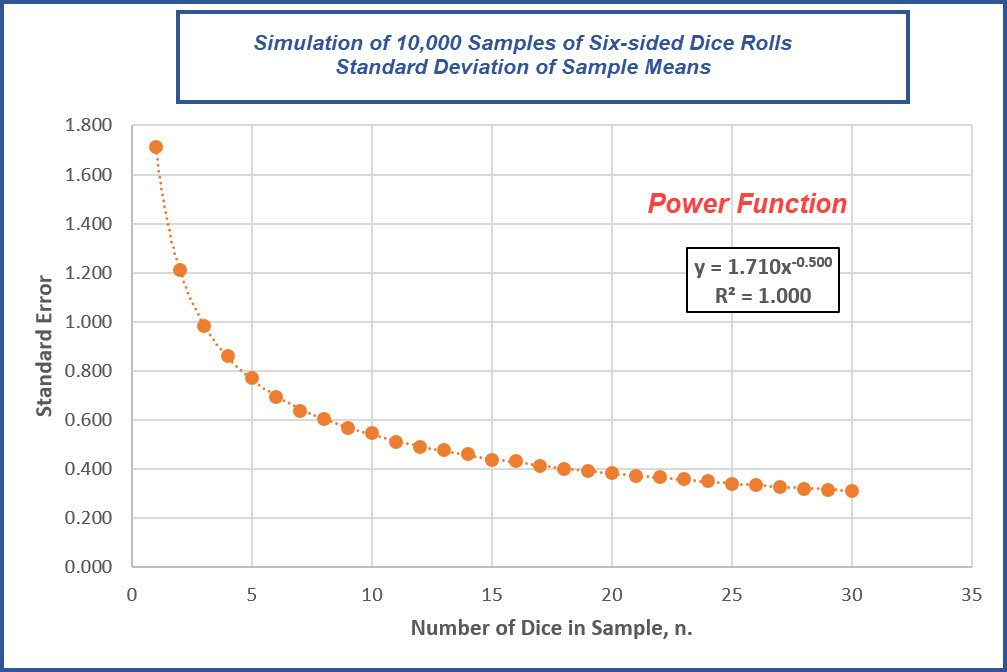Fig. #12 Of the options available in this Excel tool, the best “best fit” line to my eyes is called a “Power” function and this next image is the graph showing that trend line which is shown in Figure 13. The Power function Excel found to best fit our data does look pretty good as our 30 data points fall “precisely” on the calculated trend line. Excel gives this power line equation an R2 of 1.000, though it is not really exactly 1.000 as there are likely digits we do not see. But it is close enough that Excel rounds to 1.000, which means the equation does an excellent job of predicting the standard error given the sample size n. If I continued to simulate the standard errors for sample sizes greater than 30, they too would plot almost exactly on this power line. A Power function is an equation where the value of y is a function of x raised to a power. In this case, y is equal to 1.710 multiplied by x to the -0.5 power.Recall from algebra, a negative coefficient just means we can put x in the denominator and get a positive coefficient,Raising something to the 0.5 power is the same as taking the square root. Then, this best fit line’s equation is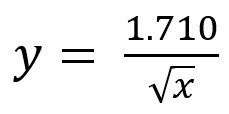This 1.710 is very, very close to the actual value of 1.7078 for the population standard deviation, σ, found earlier. If I had run even more than 10,000 samples, it is likely the numerator would have converged on sigma. Since x is our sample size, n, we have essentially shown that the sample standard error isAgain, in this exercise, I used simulations, a lot of them granted, to approximate the standard errors used by Excel to calculate the power function equation. Use this practical explanation to supplement the more precise algebraic proofs. I hope this gives you a better feeling when your instructor of textbook tells you the “proof” of why we divide sigma by the square root of the sample size n is beyond the scope of your course.

### 3 thoughts on “Why is the Standard Error Equal to Sigma Divided by the Square Root of n?”

1.Wonderful explanation, thank you!

2.Amazing explanation!!

3.Thanks! This gives me an intuitive view

This site uses Akismet to reduce spam. Learn how your comment data is processed.

Scroll to Top